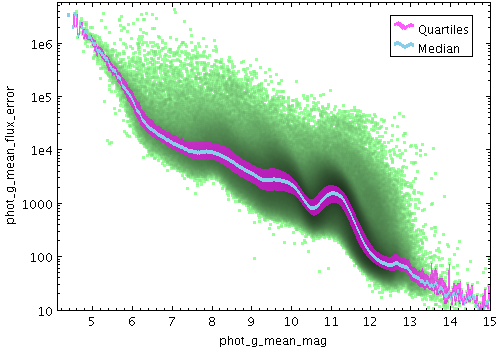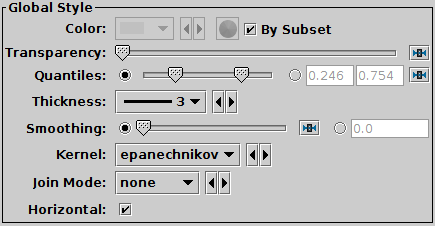Next Previous Up Contents
Next: Label Form
Up: Plot Forms
Previous: Linear Fit Form

#### A.4.5.15 Quantile Form

The Quantile form () plots a line through a given quantile of the values binned within each pixel column (or row) of a plot. The line is optionally smoothed using a configurable kernel and width, to even out noise from the pixel binning. Instead of a simple line through a given quantile, it is also possible to fill the region between two quantiles.

One way to use this is to draw a line estimating a function y=f(x) (or x=g(y)) sampled by a noisy set of data points in two dimensions.Example Quantile plotQuantile configuration panel

The configuration options are:

Transparency
Transparency with which components are plotted, from opaque to invisible.
Quantiles
Defines the quantile or quantile range of values that should be marked in each pixel column (or row). The slider control goes from 0 (minimum in pixel column/row) to 1 (maximum in pixel column/row), so 0.5 indicates the median. This control is a double-slider, so you can drag out a range of values. If the values are the same as each other, a single point will be indicated, but if there is a range then the area between the indicated quantiles will be filled.

The radio buttons let you toggle between using the slider to set the quantile value(s) or entering them in the text fields. If the two values are identical, you can leave the second text field blank.

Thickness
Sets the minimum extent of the markers that are plotted in each pixel column (or row) to indicate the designated value range. If the range is zero sized (two bounding quantiles are equal) this will give the actual thickness of the plotted line. If the range is non-zero however, the line may be thicker than this in places according to the quantile positions.
Smoothing
Configures the smoothing width. This is the characteristic width of the Kernel function to be convolved with the density in one dimension to smooth the quantile function.

You can adjust it using the slider (wider smoothing to the right) or enter a value in data coordinates explicitly in the text field. If the smoothed axis is logarithmic, the value is a multiplication factor rather than an additive increment.

Kernel
The functional form of the smoothing kernel. The functions listed refer to the unscaled shape; all kernels are normalised to give a total area of unity.

The available options are:

• square: Uniform value: f(x)=1, |x|=0..1
• linear: Triangle: f(x)=1-|x|, |x|=0..1
• epanechnikov: Parabola: f(x)=1-x*x, |x|=0..1
• cos: Cosine: f(x)=cos(x*pi/2), |x|=0..1
• cos2: Cosine squared: f(x)=cos^2(x*pi/2), |x|=0..1
• gauss3: Gaussian truncated at 3.0 sigma: f(x)=exp(-x*x/2), |x|=0..3
• gauss6: Gaussian truncated at 6.0 sigma: f(x)=exp(-x*x/2), |x|=0..6

Horizontal
Determines whether the trace bins are horizontal or vertical. If true, there is a y value calculated for each pixel column, and if false, there is an x value for each pixel row.

Next Previous Up Contents
Next: Label Form
Up: Plot Forms
Previous: Linear Fit Form

TOPCAT - Tool for OPerations on Catalogues And Tables